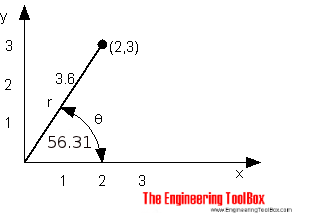Engineering ToolBox - Resources, Tools and Basic Information for Engineering and Design of Technical Applications!

# Polar vs. Cartesian Coordinates

## Convert between Cartesian and Polar coordinates.

In the Cartesian system the coordinates are perpendicular to one another with the same unit length on both axes.

Cartesian coordinates in the figure below: (2,3)A Polar coordinate system is determined by a fixed point, a origin or pole, and a zero direction or axis. Each point is determined by an angle and a distance relative to the zero axis and the origin.

Polar coordinates in the figure above: (3.6, 56.31)

Polar coordinates can be calculated from Cartesian coordinates like

r = (x2 + y2)1/2                            (1)

where

r = distance from origin to the point

x = Cartesian x-coordinate

y = Cartesian y-coordinate

θ = atan(y / x)

= tan-1(y / x)                          (2)

where

θ = angle relative to the zero axis (degrees)

Cartesian coordinates can be calculated from Polar coordinates like

x = r cos(θ)                           (3)

y = r sin(θ)                           (4)

x

y

r

θ (degrees)

## Related Topics

• Mathematics - Mathematical rules and laws - numbers, areas, volumes, exponents, trigonometric functions and more.

## Engineering ToolBox - SketchUp Extension - Online 3D modeling!

Add standard and customized parametric components - like flange beams, lumbers, piping, stairs and more - to your Sketchup model with the Engineering ToolBox - SketchUp Extension - enabled for use with the amazing, fun and free SketchUp Make and SketchUp Pro .Add the Engineering ToolBox extension to your SketchUp from the SketchUp Pro Sketchup Extension Warehouse!

Translate

## Privacy

We don't collect information from our users. Only emails and answers are saved in our archive. Cookies are only used in the browser to improve user experience.

Some of our calculators and applications let you save application data to your local computer. These applications will - due to browser restrictions - send data between your browser and our server. We don't save this data.

## Citation

• Engineering ToolBox, (2009). Polar vs. Cartesian Coordinates. [online] Available at: https://www.engineeringtoolbox.com/converting-cartesian-polar-coordinates-d_1347.html [Accessed Day Mo. Year].

Modify access date.

. .

#### Scientific Online Calculator6 24